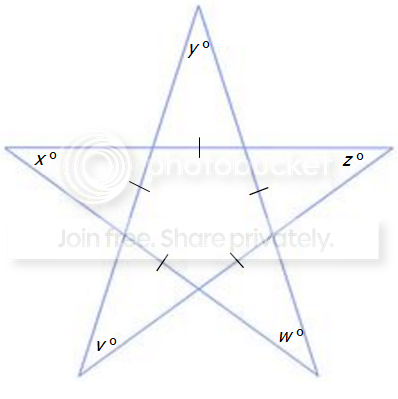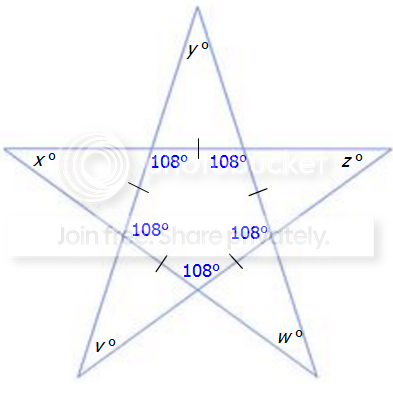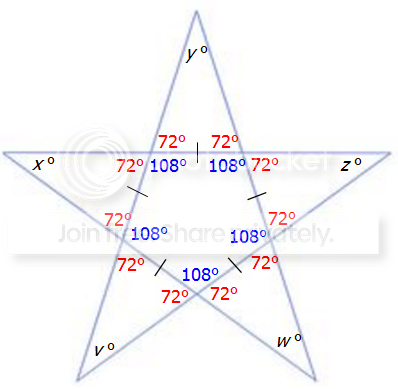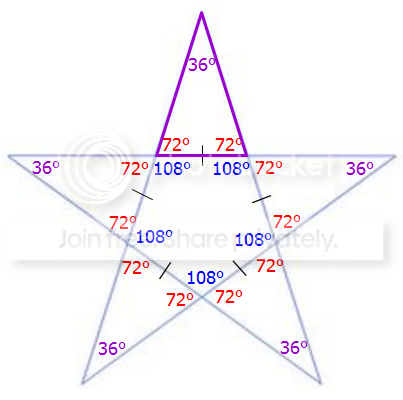GMAT Question of the Day: Daily via email | Daily via Instagram New to GMAT Club? Watch this Video

 It is currently 29 Mar 2020, 08:25### GMAT Club Daily Prep

#### Thank you for using the timer - this advanced tool can estimate your performance and suggest more practice questions. We have subscribed you to Daily Prep Questions via email.

Customized
for You

we will pick new questions that match your level based on your Timer History

Track

every week, we’ll send you an estimated GMAT score based on your performance

Practice
Pays

we will pick new questions that match your level based on your Timer History

#### Not interested in getting valuable practice questions and articles delivered to your email? No problem, unsubscribe here.# In the figure shown, what is the value of v+x+y+z+w?

Author Message
TAGS:

### Hide Tags

Math ExpertV
Joined: 02 Sep 2009
Posts: 62291
In the figure shown, what is the value of v+x+y+z+w?  [#permalink]

### Show Tags

23
15900:00

Difficulty:75% (hard)

Question Stats:59% (02:09) correct41% (02:00) wrongbased on 2216 sessions

### HideShow timer Statistics

The Official Guide for GMAT® Review, 13th Edition - Quantitative Questions Project

In the figure shown, what is the value of v+x+y+z+w?(A) 45
(B) 90
(C) 180
(D) 270
(E) 360

Diagnostic Test
Question: 10
Page: 21
Difficulty: 650

GMAT Club is introducing a new project: The Official Guide for GMAT® Review, 13th Edition - Quantitative Questions Project

Each week we'll be posting several questions from The Official Guide for GMAT® Review, 13th Edition and then after couple of days we'll provide Official Answer (OA) to them along with a slution.

We'll be glad if you participate in development of this project:
2. Please vote for the best solutions by pressing Kudos button;
3. Please vote for the questions themselves by pressing Kudos button;
4. Please share your views on difficulty level of the questions, so that we have most precise evaluation.

Thank you!

Attachment:Star.png [ 9.16 KiB | Viewed 89432 times ]

_________________
Math ExpertV
Joined: 02 Sep 2009
Posts: 62291
Re: In the figure shown, what is the value of v+x+y+z+w?  [#permalink]

### Show Tags

52
36
SOLUTION

In the figure shown, what is the value of v+x+y+z+w?(A) 45
(B) 90
(C) 180
(D) 270
(E) 360

Let's simplify the problem by imagining that we have a star that is inscribed in a circle as shown below:As we can see, 5 arcs subtended by the inscribed angles (x, y, z, w, and v) make the whole circumference. Hence the sum of the corresponding central angles must be 360 degrees, which makes the sum of the inscribed angels 360/2=180 degrees (according to the Central Angle Theorem the measure of inscribed angle is always half the measure of the central angle).

_________________
DirectorJoined: 29 Nov 2012
Posts: 675
Re: In the figure shown, what is the value of v+x+y+z+w?  [#permalink]

### Show Tags

67
1
11
I was thinking of using the exterior angle property with 2 triangles

Angle 1 = X + W
Angle 2 = v + Y

angle 1 + angle 2 + Z = 180

then add those angles up its 180 degrees.
AttachmentsStar2.jpg [ 24.67 KiB | Viewed 76435 times ]

##### General Discussion
DirectorJoined: 22 Mar 2011
Posts: 581
WE: Science (Education)
Re: In the figure shown, what is the value of v+x+y+z+w?  [#permalink]

### Show Tags

11
1
4
Bunuel wrote:
The Official Guide for GMAT® Review, 13th Edition - Quantitative Questions Project

In the figure shown, what is the value of v+x+y+z+w?
Attachment:
The attachment Star.png is no longer available

(A) 45
(B) 90
(C) 180
(D) 270
(E) 360

Diagnostic Test
Question: 10
Page: 21
Difficulty: 650

GMAT Club is introducing a new project: The Official Guide for GMAT® Review, 13th Edition - Quantitative Questions Project

Each week we'll be posting several questions from The Official Guide for GMAT® Review, 13th Edition and then after couple of days we'll provide Official Answer (OA) to them along with a slution.

We'll be glad if you participate in development of this project:
2. Please vote for the best solutions by pressing Kudos button;
3. Please vote for the questions themselves by pressing Kudos button;
4. Please share your views on difficulty level of the questions, so that we have most precise evaluation.

Thank you!

I can suggest two solutions:

Solution A

We can compute the sum of the angles from the five triangles created on the sides of the pentagon ABCDE. In those triangles, we have five pairs of congruent angles (see them marked by colored arcs in the attached drawing). Those angles are external angles for the pentagon and their sum is $$360^o$$. See at the end of the post the justification for the fact that in every convex polygon, the sum of the external angles is $$360^o$$.
Therefore, v + x + y + z + w = 5 ∙ 180 – 2 ∙ 360 = 900 – 720= 180.

Solution B

Since the question is a multiple choice one, we can assume that there is one correct answer and that that answer does not depend on the shape of the “star”. Assuming that the star can be inscribed in a circle, we can see that the requested sum of the angles is 360/2 = 180, because each angle is inscribed in the circle and the five corresponding arcs complete the circle.
Remark: If one of the answers would have been “It cannot be determined” or something similar, than this argument wouldn’t work.

Sum of the external angles for a convex polygon:

We know that the sum of the interior angles in a convex polygon with n sides (n being a positive integer greater than 2) is given by the formula:
(n – 2)∙180 = 180n – 360.
Each external angle is 180 – the corresponding interior angle. Therefore, the total sum of the exterior angles is 180n – (n – 2) ∙ 180 = 180n – 180n + 360 = 360.

Note: Convex polygons have the property that each of their angles is less than 180. All the polygons dealt with on GMAT are convex (triangle, quadrilateral, pentagon, hexagon,...) or are made up of convex polygons. In this question, the figure of the star, without the sides of the small convex pentagon, is an example of a non-convex decagon: it has 10 sides, and 5 angles which are greater, and 5, which are smaller than 180.
AttachmentsOG13-D10-A.png [ 26.98 KiB | Viewed 78760 times ]OG13-D10-B.png [ 38.38 KiB | Viewed 78339 times ]

_________________
PhD in Applied Mathematics
Love GMAT Quant questions and running.
Senior ManagerB
Joined: 28 Mar 2012
Posts: 292
Location: India
GMAT 1: 640 Q50 V26
GMAT 2: 660 Q50 V28
GMAT 3: 730 Q50 V38
Re: In the figure shown, what is the value of v+x+y+z+w?  [#permalink]

### Show Tags

26
9
Hi,

With reference to the attached diagram:
In triangle DFB, angle DFB = 180 - angle FDB - angle FBD
=180 - v - z

Similary, in other triangles,
angle AJC = 180 - y - w
angle EIB = 180 - x - z
angle AHD = 180 - v - y
angle EGC = 180 - x - w

Adding all, angle DFB + angle AJC + angle EIB + angle AHD +angle EGC = sum of interior angles of pentagon
=540 = 5*180 - 2(v+w+x+y+z)
so, v+w+x+y+z = 180

Regards,
Attachmentsangles.jpg [ 12.75 KiB | Viewed 78199 times ]

Originally posted by cyberjadugar on 25 Jun 2012, 03:23.
Last edited by cyberjadugar on 25 Jun 2012, 04:09, edited 1 time in total.
Math ExpertV
Joined: 02 Sep 2009
Posts: 62291
In the figure shown, what is the value of v+x+y+z+w?  [#permalink]

### Show Tags

2
1
SOLUTION

In the figure shown, what is the value of v+x+y+z+w?(A) 45
(B) 90
(C) 180
(D) 270
(E) 360

Let's simplify the problem by imagining that we have a star that is inscribed in a circle as shown below:As we can see, 5 arcs subtended by the inscribed angles (x, y, z, w, and v) make the whole circumference. Hence the sum of the corresponding central angles must be 360 degrees, which makes the sum of the inscribed angels 360/2=180 degrees (according to the Central Angle Theorem the measure of inscribed angle is always half the measure of the central angle).

Attachment:Star2.png [ 43.64 KiB | Viewed 91862 times ]

Attachment:Star.png [ 9.16 KiB | Viewed 90624 times ]

_________________
ManagerJoined: 28 Sep 2011
Posts: 56
Location: United States
GMAT 1: 520 Q34 V27
GMAT 3: 690 Q47 V38
GPA: 3.01
WE: Information Technology (Commercial Banking)
Re: In the figure shown, what is the value of v+x+y+z+w?  [#permalink]

### Show Tags

2
The sum of all the angles of the pentagon in the middle is (5-3)180 = 540.
Each vertex of the pentagon has a angle of 108 degrees (on average).
Lets look at triangle AYW, since A is a vertex of the pentagon, the sum of y + w = 72.
In this same scenario z + v also = 72 and x equals 36 (72/2).

72 + 72 + 36 = 180
DirectorJoined: 22 Mar 2011
Posts: 581
WE: Science (Education)
Re: In the figure shown, what is the value of v+x+y+z+w?  [#permalink]

### Show Tags

ashish8 wrote:
The sum of all the angles of the pentagon in the middle is (5-3)180 = 540.
Each vertex of the pentagon has a angle of 108 degrees (on average).
Lets look at triangle AYW, since A is a vertex of the pentagon, the sum of y + w = 72.
In this same scenario z + v also = 72 and x equals 36 (72/2).

72 + 72 + 36 = 180

Nice! In other words, if we understand from the answers that the sum does not depend on the shape of the star, we can consider a regular pentagon. Then each of its angles is 108, and each angle of the star is 36, so the sum is 180.
_________________
PhD in Applied Mathematics
Love GMAT Quant questions and running.
InternJoined: 03 Feb 2011
Posts: 13
Re: In the figure shown, what is the value of v+x+y+z+w?  [#permalink]

### Show Tags

Hi Bunuel,

I didn't understand the part where you used "Central Angle Theorem". Is it correct to assume that if I see one or more triangles then If I draw a
circle around those triangles which are kind of inter-connected then can I use the "Central Angle Theorem"?

Thanks a lot for the help,

LR
Math ExpertV
Joined: 02 Sep 2009
Posts: 62291
Re: In the figure shown, what is the value of v+x+y+z+w?  [#permalink]

### Show Tags

5
nitestr wrote:
Hi Bunuel,

I didn't understand the part where you used "Central Angle Theorem". Is it correct to assume that if I see one or more triangles then If I draw a
circle around those triangles which are kind of inter-connected then can I use the "Central Angle Theorem"?

Thanks a lot for the help,

LR

Not sure understand what you mean.

Anyway, the Central Angle Theorem states that the measure of inscribed angle is always half the measure of the central angle:For more check here: math-circles-87957.html
_________________
InternJoined: 03 Feb 2011
Posts: 13
Re: In the figure shown, what is the value of v+x+y+z+w?  [#permalink]

### Show Tags

I'm ok with the Theorem, but I want to know how you came up the assumption " Let's simplify the problem by imagining that we have a star that is inscribed in a circle as shown below" as you mentioned above?
ManagerJoined: 18 Aug 2006
Posts: 80
Location: United States
WE: Consulting (Telecommunications)
Re: In the figure shown, what is the value of v+x+y+z+w?  [#permalink]

### Show Tags

1
nitestr wrote:
I'm ok with the Theorem, but I want to know how you came up the assumption " Let's simplify the problem by imagining that we have a star that is inscribed in a circle as shown below" as you mentioned above?

You are right, not always can we conclude that five points in a plan are co-circular; while it is true that three points are always co-circular.

However, the solution works if we imagine two circles for three points each. It was just a coincidence that the assumption that all the given points are co-circular worked.
InternJoined: 19 Jan 2014
Posts: 23
GMAT 1: 730 Q50 V39
WE: Accounting (Accounting)
Re: In the figure shown, what is the value of v+x+y+z+w?  [#permalink]

### Show Tags

1
Attachment:star-sol.png [ 10.08 KiB | Viewed 37779 times ]

i didn't know the central angle theorem, and tried solving with a different way.
sorry for my mad paint skills :D

here is my solution:
1. Draw a line via vertex of angle Y, parallel to the line between angles V and Z. In a picture the red coloured lines are parallel.
2. Draw a line via vertex of angle Y, parallel to the line between angles X and Z (violet coloured)
3. Draw a line via vertex of angle Y, parallel to the line between angles X and W (blue coloured)

The following can be concluded from the pic accoridng to thales theorem:
a. angles between red and violet lines will be same (angle Z)
b. angles between black and red lines will be same (angle V)
c. angles between blue and black lines will be same (angle W)
d. angles between blue and violet lines will be same (angle X)

as a result sum of 5 angles will be a violet line and equal to 180 degrees

My solution is obviously not as simple and quick as Bunuel's one, but maybe you can use my approach for solving similar problems
Senior ManagerB
Joined: 04 Jul 2014
Posts: 293
Location: India
GMAT 1: 640 Q47 V31
GMAT 2: 640 Q44 V34
GMAT 3: 710 Q49 V37
GPA: 3.58
WE: Analyst (Accounting)
Re: In the figure shown, what is the value of v+x+y+z+w?  [#permalink]

### Show Tags

Bunuel wrote:
Let's simplify the problem by imagining that we have a star that is inscribed in a circle as shown below:Hi Bunuel. How can we assume that the star could be inscribed within a circle in the absence of information. Further, the general GMAT assumption is that all diagrams are not drawn to scale unless the contrary is mentioned.

Am I missing anything here? Please help!Senior ManagerB
Joined: 04 Jul 2014
Posts: 293
Location: India
GMAT 1: 640 Q47 V31
GMAT 2: 640 Q44 V34
GMAT 3: 710 Q49 V37
GPA: 3.58
WE: Analyst (Accounting)
Re: In the figure shown, what is the value of v+x+y+z+w?  [#permalink]

### Show Tags

5
5
Wow! See what I found with a little googling!A star is always regularly shaped (this clarifies why Bunuel assumed that the start would get inscribed in the circle)!
1. The sum of the angles formed at the tips of the five pointed star is 180; the sum of the angles formed at the tips of the six pointed star is 360.
2. The formula for the sum of the angle measurements at the tips of an n-pointed star is f(n)=180(n)-720 where n is an integer greater than 4.

Read more here: http://mathforum.org/pom2/nov.98/winner.htmlThis clarifies my doubts Very Happy
InternJoined: 21 Jul 2015
Posts: 34
Re: In the figure shown, what is the value of v+x+y+z+w?  [#permalink]

### Show Tags

3
1
Interior angle of a polygon = $$((n-2)180)/n$$
For Pentagon = $$(5-3)180/5$$ = 108
sum of angles in each triangle angle x+(180-108)+(180-108) = 180 .... x = 36
there are 5 triangles ... 36*5 = 180
InternB
Joined: 16 Sep 2015
Posts: 9
Re: In the figure shown, what is the value of v+x+y+z+w?  [#permalink]

### Show Tags

Alternate Solution with just the basics.
(I have not named every vertex separately in order to avoid confusion (mess). Every vertex of the star is named by the angle it depicts in the picture)
Attachments

File comment: y+A+B+C = 360 (sum of all the angles in quadrilateral-YABC )
A= 180-(y+v) (sum of all angles in a triangle is 180,Triangle YAV)
B= 180-(x+z) (Triangle XBZ)
C= 180-(y+w) (Triangle YCW)

Now, Substitute the values of A, B and C in the equation : A+B+C+y=360
(180-y-v) + (180-x-z) + (180-y-w) + y =360
By solving the above, we get:
540 - w - v - z - x - 2y + y=360
x+y+v+z+w=180

Govind Kohli
Kudos if this helpsstep0004.png [ 5.55 KiB | Viewed 36840 times ]

Senior ManagerB
Joined: 23 Sep 2015
Posts: 368
Location: France
GMAT 1: 690 Q47 V38
GMAT 2: 700 Q48 V38
WE: Real Estate (Mutual Funds and Brokerage)
Re: In the figure shown, what is the value of v+x+y+z+w?  [#permalink]

### Show Tags

6
2
$$A + B + C + D + E = (5-2)*180 = 540$$

$$A+x+z=180$$
$$B+y+v=180$$
$$C+x+w=180$$
$$D+v+z=180$$
$$E+y+w=180$$

$$A + B + C + D + E +2x+2y+2z+2w+2v =900$$

$$540 + 2x+2y+2z+2w+2v = 900$$

$$2x+2y+2z+2w+2v = 360$$

$$x+y+z+w+v=180$$
AttachmentsGMAT.png [ 8.59 KiB | Viewed 36955 times ]

_________________
GMAT Club LegendV
Joined: 11 Sep 2015
Posts: 4547
GMAT 1: 770 Q49 V46
Re: In the figure shown, what is the value of v+x+y+z+w?  [#permalink]

### Show Tags

20
Top Contributor
4
Bunuel wrote:
The Official Guide for GMAT® Review, 13th Edition - Quantitative Questions Project

In the figure shown, what is the value of v+x+y+z+w?(A) 45
(B) 90
(C) 180
(D) 270
(E) 360

First, let's consider a PERFECT star.Notice that the pentagon in the center is a perfect (regular) pentagon, which means ALL 5 angles are equal.
The sum of the angles in an n-sided figure = 180(n-2) degrees
So, the sum of the angles in this 5-sided figure = 180(5-2) = 180(3) = 540 degrees
Since ALL 5 angles are equal, then the measure of each angle = 540/5 = 108 degrees.Since two angles on a line must add to 180 degrees, we can see that the angles adjacent to the 108-degree angles must equal 72 degrees (since 180 - 108 = 72)At this point, we can see that we're dealing with 5 triangles, and for each triangle, we know two of the angle measurements.
Since the sum of the angles in a triangle = 180, we know that each missing angle = 36 degrees (180 - 72 - 72 = 36)So, v + x + y + z + w = 36 + 36 + 36 + 36 + 36
= 180 degrees

_________________
InternB
Joined: 13 Apr 2017
Posts: 4
Location: Portugal
Concentration: General Management, Economics
WE: General Management (Retail)
In the figure shown, what is the value of v+x+y+z+w?  [#permalink]

### Show Tags

2
Although GMAT pictures may be not completely accurate and drawn to scale, I realize they don't usually try to "trick" users... Therefore I just drew the figure on a piece of paper and given the answer choices I realized the sum of the angles must be somewhere around 180 degrees:
Attachment:star.png [ 31.16 KiB | Viewed 35930 times ]

Probably this approach is not the most scientific and reliable way to solve the problem, but I guess it worked on this one.In the figure shown, what is the value of v+x+y+z+w?   [#permalink] 30 Jul 2017, 03:44

Go to page    1   2    Next  [ 24 posts ]

Display posts from previous: Sort by

# In the figure shown, what is the value of v+x+y+z+w?